[BACK]
 Intelligent Automation & Soft ComputingDOI:10.32604/iasc.2021.014962Article

Short-Term Stock Price Forecasting Based on an SVD-LSTM Model

1Department of Finance and Taxation, Shandong University of Finance and Economics, Jinan, 250014, China
2Department of Computer science and Technology, Shandong University of Finance and Economics, Jinan, 250014, China
*Corresponding Author: Peiguang Lin. Email: llpwgh@163.com
Received: 29 October 2020; Accepted: 04 February 2021

Abstract: Stocks are the key components of most investment portfolios. The accurate forecasting of stock prices can help investors and investment brokerage firms make profits or reduce losses. However, stock forecasting is complex because of the intrinsic features of stock data, such as nonlinearity, long-term dependency, and volatility. Moreover, stock prices are affected by multiple factors. Various studies in this field have proposed ways to improve prediction accuracy. However, not all of the proposed features are valid, and there is often noise in the features—such as political, economic, and legal factors—which can lead to poor prediction results. To overcome such limitations, this study proposes a forecasting model for predicting stock prices in a short-term time series. First, we use singular value decomposition (SVD) to reconstruct the features of stock data, eliminate data noise, retain the most effective data features, and improve the accuracy of prediction. We then model the time-series stock data based on a long short-term memory (LSTM) model. We compare our proposed SVD-LSTM model with four state-of-the-art methods using real-world stock datasets from two Chinese banks: Ping an Bank and Shanghai Pudong Development Bank. The experimental results show that the proposed method can improve the accuracy of stock price predictions.

Keywords: Short-term stock price forecasting; singular value decomposition; deep learning

1  Introduction

As a high-risk but high-yield investment method, stock trading has received a great deal of attention from both investors and researchers. However, predicting stock prices and stock movement is challenging because of uncertainties such as political factors, market factors, and environmental factors.

Since stock prices are affected by multiple factors, researchers have introduced various features to improve the results of stock price prediction. However, there is often noise in these features, which affects the accuracy of stock price prediction. Moreover, traditional forecasting methods typically use statistical models to make predictions based on linear connections between stocks. Yet, given the existence of nonlinearity in stock data, these types of statistical models often fail to accurately predict stock prices. To address this problem, the present study developed a model based on singular value decomposition (SVD) and long short-term memory (LSTM).

SVD, as a matrix decomposition method, has been used extensively in the imaging field. For example, SVD can be used to compress images by reconstructing an image matrix based on singular values . In recent years, with the development of artificial neural networks (ANNs) , LSTM networks have facilitated significant progress in research on processing time-series data . Compared to traditional multilayer perceptron (MLP) , convolutional neural network (CNN) , and recurrent neural network (RNN) models, LSTM networks account for the long-term nature of time-series stock data and add three gates to deal with problems such as vanishing or exploding gradients.

Stock prices are highly prone to volatility as a result of political and economic factors, among others. For this study, we used the top 30% of forecasting results as short-term forecasts, and all forecast results were used as long-term forecasts. The experimental results indicated that our proposed model achieved better results for short-term prediction than for long-term prediction.

This study proposes a deep learning model for predicting stock prices in a time series based on an SVD-LSTM model. We used SVD to reconstruct the data by selecting partial features with large singular values; this can eliminate noise in the data and improve data quality. Meanwhile, LSTM was used to train the cleaned input data and predict the closing prices of stocks. In our experiments, the proposed SVD-LSTM model was shown to outperform MLP, CNN, LSTM, and PCA-LSTM models in the short-term prediction of stock prices.

2  Related Work

There are many well-known models for stock forecasting, such as the autoregressive (AR) model, autoregressive–moving-average (ARMA) model, and autoregressive integrated moving average (ARIMA) model [6,7]. These traditional time-series stock models mainly rely on linear dependency among stock prices. In reality, however, such linearity does not apply to time series because of factors such as the political climate, and traditional time-series models thus have difficulty predicting stock prices with acceptable accuracy.

With the development of neural networks, Bayesian [8,9] and decision tree  models, among others, have been used for time-series forecasting. However, such models have difficulty accurately predicting stock prices since they are primarily suited for classification tasks. Given their successful application in the field of image processing, CNN models were subsequently adopted for predicting time-series data. For example, using a stock dataset consisting of 1721 companies listed on the National Stock Exchange of India, Selvin et al.  was able to accurately predict stock prices using a CNN model.

Nevertheless, CNN models are mainly applied in the field of image processing through operations such as convolution. While CNN models are used to retain image features and reduce the search space of image processing, they cannot fully consider the temporal dependency of stock prices. For example, using an eight-year stock dataset from the Chinese company Pingtan, Li et al.  found that RNN models predicted stocks more accurately than certain traditional machine learning models. However, when handling data with a long time sequence, RNN models are prone to problems such as vanishing or exploding gradients, which reduce prediction accuracy. To address such problems, Hochreiter and Schmidhuber  proposed LSTM—a variant of RNN—which comprises three control units: a forget gate, an input gate, and an output gate. Using an LSTM model to predict the stock price of Chinese pharmaceutical company Yunnan Baiyao, Wang et al.  achieved a prediction accuracy of 60–65%. In the present study, therefore, we also used an LSTM model to forecast stock prices.

Stock data have many different characteristics, each of which has a different effect (weight) on price forecasting. It is important, then, that stock prediction models take such characteristics into consideration.

The traditional processing method of principal component analysis (PCA) reduces the dimensionality of stock data by only considering the most representative data features. In SVD, however, PCA is mainly applied to the diagonal and right singular matrix and is not applicable to processing the left singular matrix. Han  achieved good prediction results in a time series by leveraging a newly proposed SVD-based time-series neural network. Thus, in our study, we used an SVD model to reconstruct stock data in the feature-processing stage, which helped to clean up noise in the data.

We should note that, in SVD, large singular values indicate influential information while small singular values refer to noisy information. In this study, we considered only large singular values when reconstructing the data and ignored small ones with noise. We reconstructed the data matrix using singular values whose accumulated weights accounted for more than 90% of all singular values.

3  Time-Series Stock Forecasting Model Based on SVD-LSTM

This section describes using SVD to process the data and then using LSTM as the prediction model.

3.1 Data Preprocessing

Stock data involve many characteristics, such as closing price, opening price, highest price, lowest price, and transaction value. Using the closing price as the predicted value, we employed the SVD method to retrieve influential factors (e.g., opening price) that are closely related to the predicted value and then reconstructed the input matrix by eliminating noise in the data.

3.1.1 Data Standardization

Given the different scales of stock characteristics, we standardize these characteristics via Eq. (1):

x~=xxminxmaxxmin (1)

3.1.2 Singular Value Decomposition

SVD decomposition is essentially a type of matrix decomposition. For stock data, it can be represented as a matrix of m×n , where m is the number of stock data records and n is the number of stock features (i.e., dimensionality). In this study, n represents features other than the stock closing price:

X=(x11x1nxm1xmn) (2)

SVD is used to decompose the stock data matrix as follows:

X=UΣVT (3)

XTX=(VSTUT)USVT=V(STS)VT (4)

XXT=USVT(VSTUT)=U(SST)UT (5)

σi=λi (6)

In Eq. (4), the feature matrix XTX is actually the V matrix in SVD. In Eq. (5), the feature matrix XXT is actually the U matrix in SVD. In Eq. (6), the square root of the eigenvalue of the Σ matrix is equal to the eigenvalue of the XTX matrix. Specifically, matrix U is a right singular matrix of m×m , matrix Σ is a matrix of m×n , and matrix V is an n×n right singular matrix.

Based on SVD, matrix X can be converted into a form in which three matrices are multiplied. The diagonal elements of matrix Σ are the singular values of matrix X , which can approximately reflect feature importance in the matrix. The small singular values in the matrix can be ignored since they can be considered noise.

3.2 Stock Forecasting Model

We chose an LSTM model as the prediction tool since it can handle temporal dependency in stock data. An LSTM network is a variant of an RNN; RNNs use sequence data as inputs and connect the units in chains .

An RNN model’s memory feature can save the status of previous stages and transfer it to later stages. In the training process, an RNN model can save and transmit previous inputs as a hidden state. In an RNN model, the output is generated jointly by the current input and the previously saved units.

RNNs are primarily trained via back-propagation. However, given a long-term input sequence, it is possible to lose the gradient in the process of back-propagation. To overcome this, we used an LSTM model as the training model (which, as mentioned earlier, includes forget, input, and output gates).

The forget gate (i.e., Eq. ) determines the amount of information retained from previous states, where σ is the sigmoid function, W is the weight, and b is a bias term. When input data at the current moment passes through the forget gate, the sigmoid function of the forget gate will map the input data to either 1 or 0, where 1 and 0 indicate the pass or fail of the input values, respectively.

ft=σ(Wf[ht1,xt]+bf) (7)

The input gate determines the amount of input states retained from the current state. More specifically, the input gate determines the amount of data to be retained at the current moment via Eq. (8), obtains the new candidate value C~t via Eq. (9), and updates the current cell state via Eq. (10):

it=σ(Wi[ht1,xt]+bi) (8)

C~t=tanh(WC[ht1,xt]+bC) (9)

Ct=ftCt1+itC~t (10)

The output gate determines the amount of output information based on the LSTM model via Eq. (11) and (12):

ot=σ(Wo[ht1,xt]+bo) (11)

ht=ottanh(Ct) (12)

4  Experiment

4.1 Dataset

We evaluated the performance of the proposed model using two real bank datasets—Ping an Bank and Shanghai Pudong Development Bank (SPD Bank)—collected from January 4, 2009, to December 31, 2019. For both datasets, we selected five attributes as the characteristics: opening price, closing price, highest price, lowest price, and trading volume. We used the SVD-LSTM model as the training model and set 70% of the data as the training set and the remaining 30% as the testing set. We then used the top 30% of the test dataset as the short-term prediction reference data.

4.2 Feature Extraction

Using the closing price as the prediction target, we decomposed the other data features based on SVD, reconstructed the data matrix by selecting singular values whose accumulated weights accounted for more than 90% of all singular values, and cleaned the data noise. Tab. 1 shows the singular stock values of Ping an Bank and SPD Bank. From the results, the weights of the first two singular values accounted for more than 90% in both datasets. We then reconstructed the input matrix by selecting the first two singular values.

Table 1: Singular Values for Ping An Bank and SPD Bank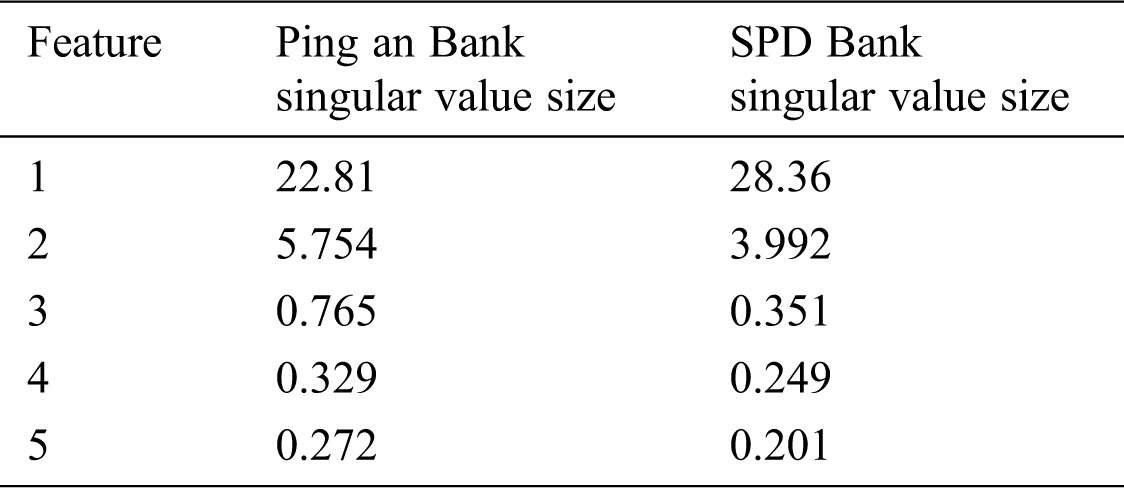4.3 Model Evaluation Indicators

We evaluated the performance of our SVD-LSTM model against four other models—MLP, CNN, LSTM, and PCA-LSTM—using three metrics: root-mean-square error (RMSE), mean absolute percent error (MAPE), and mean absolute error (MAE):

RMSE=1ni=1n(y^iyi)2 (13)

MAPE=100%ni=1n|y^iyiy| (14)

MAE=1ni=1n|y^iyi| (15)

where y^i represents the predicted value of the stock price, and yi represents the real stock price data. The smaller the values of the three metrics, the better the performance of the model; the larger the values, the poorer the performance.

4.4 Parameter Sensitivity

An LSTM model’s performance is affected by the choice of parameters. To study the sensitivity of the parameters, we simplified the analysis of the number of hidden neurons. Using other parameters as the default values, we varied the number of neurons as 16, 32, 64, 128, and 256. We selected the optimal hidden neuron parameter by determining the RMSE of all predicted datasets under different hidden neuron parameter settings.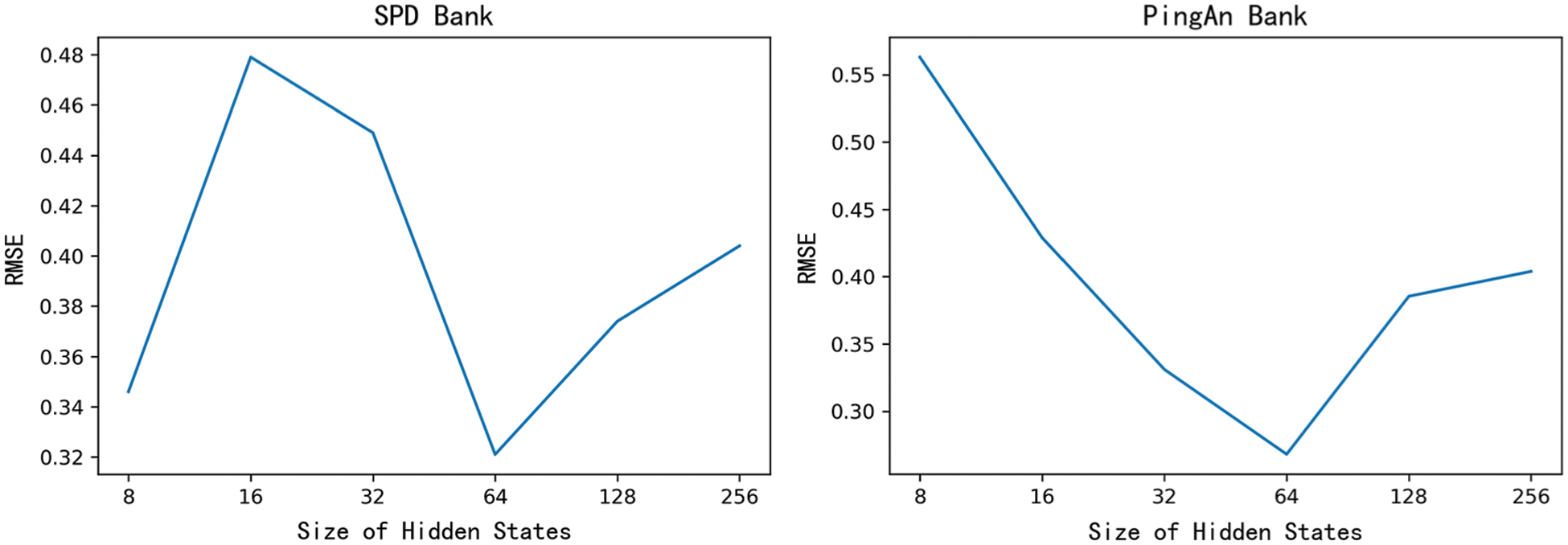Figure 1: RMSE corresponding to different hidden neurons for Ping an Bank and SPD Bank

Fig. 1 shows that the two datasets achieved the best performance (i.e., the lowest RMSE values) when the number of hidden neurons was set to 64. Therefore, we used an SVD-LSTM model with 64 hidden neurons for stock prediction.

4.5 Analysis of Results

We evaluated the performance of our SVD-LSTM model against MLP, CNN, LSTM, and PCA-LSTM models.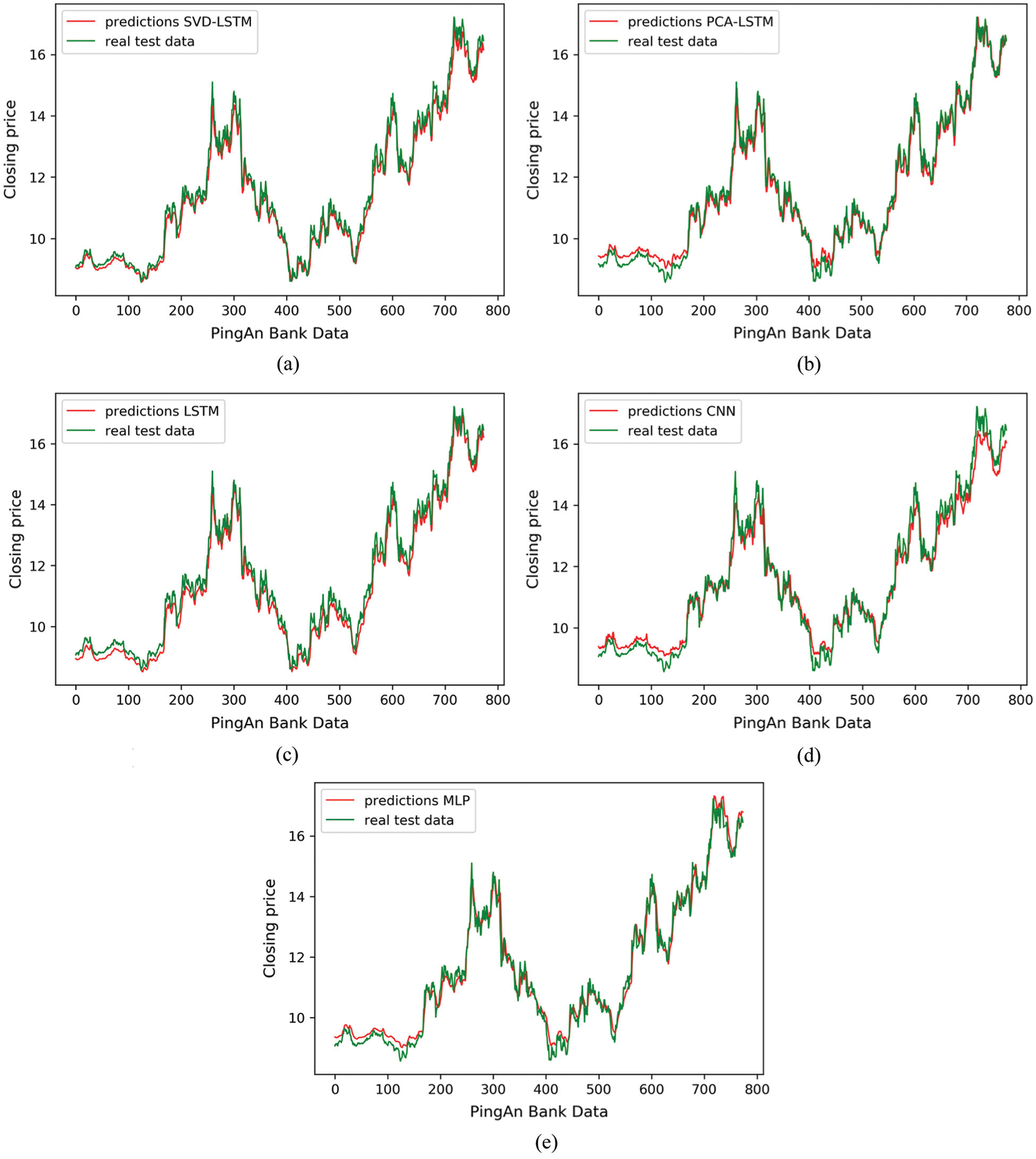Figure 2: Comparison of different models’ prediction results for Ping An Bank (a) Ping An Bank SVD-LSTM forecast (b) Ping An Bank PCA-LSTM forecast (c) Ping An Bank LSTM forecast (d) Ping An Bank CNN forecast (e) Ping An Bank MLP forecastFigure 3: Comparison of different models’ prediction results for SPD Bank (a) SPD Bank SVD-LSTM forecast (b) SPD Bank PCA-LSTM forecast (c) SPD Bank LSTM forecast (d) SPD Bank CNN forecast (e) SPD Bank MLP forecast

Table 2: Evaluation index results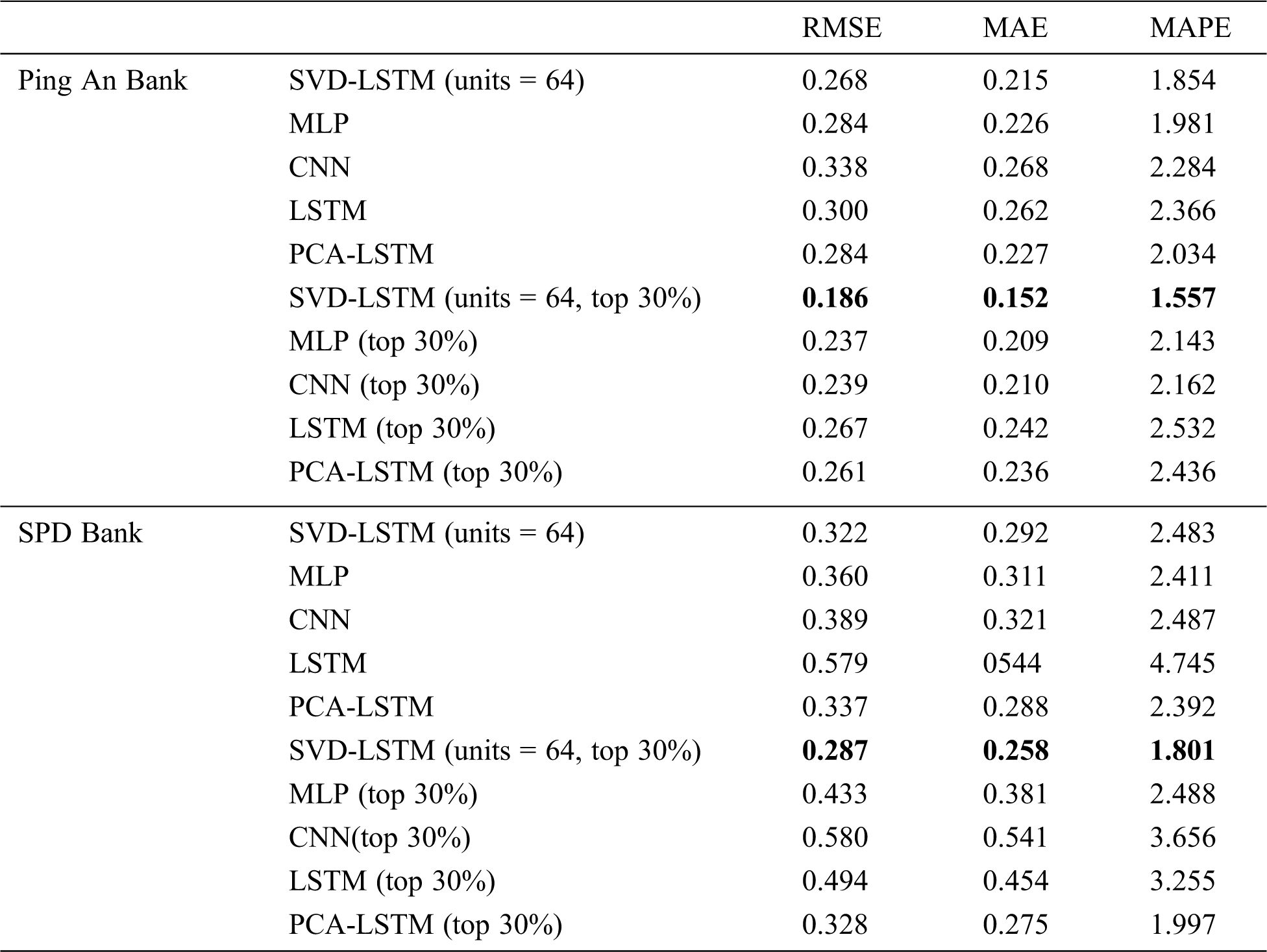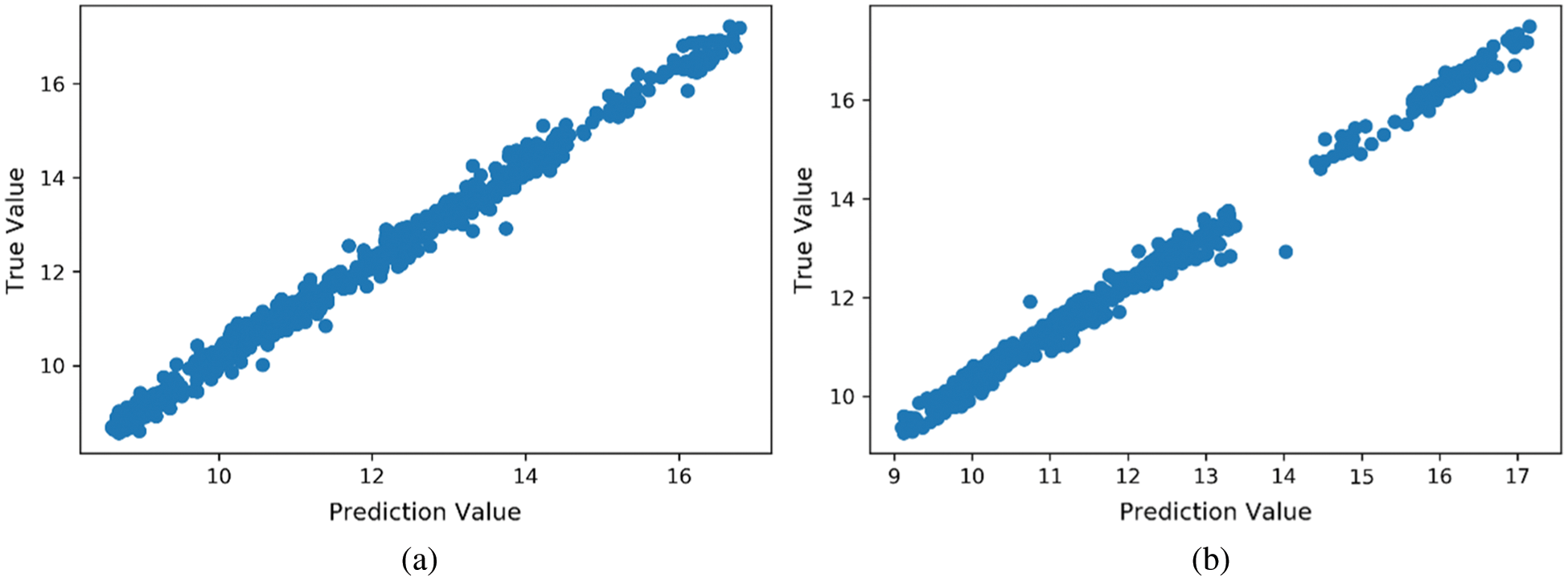Figure 4: Scatterplots of real and forecasted data for Ping an Bank and SPD Bank (a) Scatterplot of real and forecasted data for Ping An Bank (b) Scatterplot of real and forecasted data for SPD Bank

Figs. 2 and 3 show that the proposed SVD-LSTM model performed better than the other models for both datasets. Moreover, as shown in Tab. 2, our proposed SVD-LSTM model outperformed the others for both datasets with regard to the MAE, MAPE, and RMSE metrics. We can also see in Tab. 2 that our proposed SVD-LSTM model achieved higher accuracy in predicting data in the short term versus the long term, thus confirming the advantage of the SVD-LSTM model for short-term stock forecasting.

Fig. 4 shows scatterplots of the real and predicted values obtained by the SVD-LSTM model for the two banks. Ideally, the scatter points should be distributed around the straight line with a slope of 1, which is clearly the case in the figure. This verifies the predictive validity of our proposed SVD-LSTM model.

In summary, our experiments using datasets for SPD Bank and Ping an Bank verified the effectiveness of the proposed SVD-LSTM model for the short-term prediction of stock data.

5  Conclusion

This study proposed a novel SVD-LSTM model for predicting stock prices. The model uses SVD to clean data noise and reconstruct the data matrix. In our experiments using datasets for Ping an Bank and SPD Bank, the proposed SVD-LSTM model outperformed four other models (i.e., MLP, CNN, LSTM, and PCA-LSTM) in predicting short-term stock prices.

Nevertheless, we considered only four stock characteristics—opening price, highest price, lowest price, and trading volume—and ignored the influence of other factors. In future work, we will consider additional factors (e.g., emotional indicators or policy factors), seek to improve the model’s accuracy for long-term predictions, and improve the model’s performance by dynamically optimizing parameters.

Funding Statement: Project manager: Chen Zhang. Grant number: 61802230. Type of funding: National Science Foundation of China.

Conflicts of Interest: The authors declare that they have no conflicts of interest to report regarding this research.

References

1.  1.  H. Andrews and C. Patterson. (1976). “Singular value decomposition (SVD) image coding,” IEEE Trans. on Communications, vol. 24, no. 4, pp. 425–432.
2.  2.  M. Qiu and Y. Song. (2016). “Predicting the direction of stock market index movement using an optimized artificial neural network model,” PLoS One, vol. 11, no. 5, pp. e0155133.
3.  3.  F. G. Liu, M. Q. Cai, L. M. Wang and Y. S. Lu. (2019). “An ensemble model based on adaptive noise reducer and over-fitting prevention LSTM for multivariate time series forecasting,” IEEE Access, vol. 7, pp. 26102–26115.
4.  4.  M. Khashei and Z. Hajirahimi. (2018). “A comparative study of series ARIMA/MLP hybrid models for stock price forecasting,” Communication in Statistics Simulation and Computation, vol. 48, no. 9, pp. 1–16.
5.  5.  O. B. Sezer and A. M. Ozbayoglu. (2018). “Algorithmic financial trading with deep convolutional neural networks: Time series to image conversion approach,” Applied Soft Computing, vol. 70, no. 2, pp. 525–538.
6.  6.  S. S. Nie. (2012). “The historical development of time series analysis,” Journal of Guangxi University for Nationalities (Natural Science Edition), vol. 18, no. 1, pp. 24–28.
7.  7.  P. F. Pai and C. S. Lin. (2005). “A hybrid arima and support vector machines model in stock price forecasting,” Omega-Int. Journal of Management Science, vol. 33, no. 6, pp. 497–505.
8.  8.  E. Kita, M. Harada and T. Mizuno. (2012). “Application of Bayesian network to stock price prediction,” Artificial Intelligence Research, vol. 1, no. 2, pp. 171–184.
9.  9.  Y. Zuo and E. Kita. (2012). “Stock price forecast using Bayesian network,” Expert Systems with Applications, vol. 39, no. 8, pp. 6729–6737.
10. 10. T. S. Chang. (2011). “Comparative study of artificial neural networks, and decision trees for digital game content stocks price prediction,” Expert Systems with Applications, vol. 38, no. 12, pp. 14846–14851.
11. 11. S. Selvin, R. Vinayakumar and E. A. Gopalakrishna. (2017). “Stock price prediction using lstm, rnn and cnn-sliding window model,” in Proc. ICACCI, Karnataka, India, pp. 1643–1647.
12. 12. W. Li and L. Jian. (2017). “A comparative study on trend forecasting approach for stock price time series,” in Proc. ASID, Xiamen, China, pp. 74–78.
13. 13. S. Hochreiter and J. Schmidhuber. (1997). “Long short-term memory,” Neural Computation, vol. 9, no. 8, pp. 1735–1780.
14. 14. Y. Wang, Y. Liu and M. Wang. (2018). “Lstm model optimization on stock price forecasting,” in Proc. DCABES, Wuxi, pp. 173–177.
15. 15. M. Han, M. Fan and Z. Shi. (2006). “Multivariate time series prediction by neural network combining svd,” in Proc. SMC, Beijing, China, pp. 3884–3889.
16. 16. H. Sadr, M. M. Pedram and M. Teshnehlab. (2019). “A robust sentiment analysis method based on sequential combination of convolutional and recursive neural networks,” Neural Processing Letters, vol. 50, no. 3, pp. 2745–2761.This work is licensed under a Creative Commons Attribution 4.0 International License, which permits unrestricted use, distribution, and reproduction in any medium, provided the original work is properly cited.